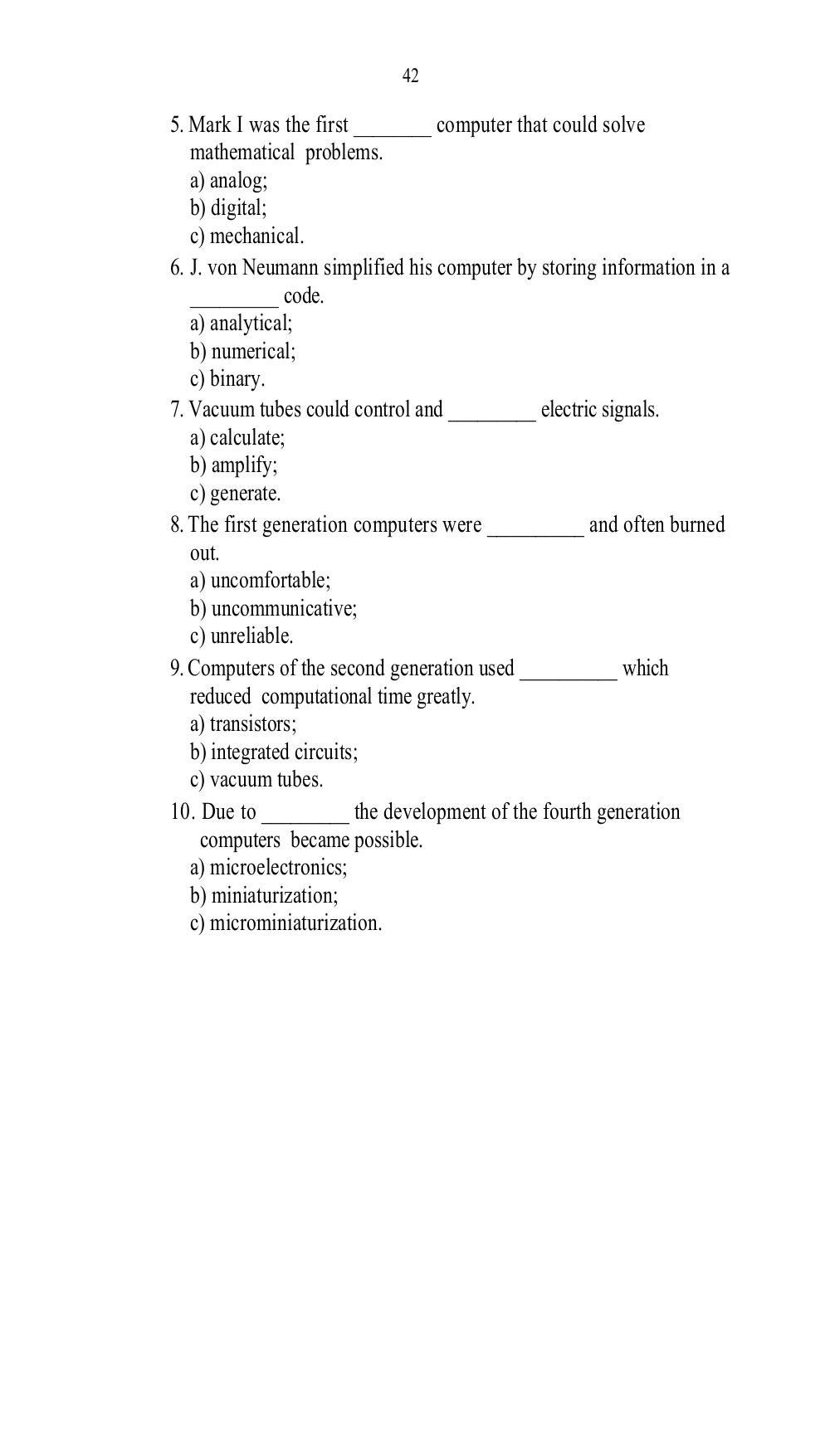# Welcome to the computer world. Practice materials for 1st year students. Еранина Т.И - 42 стр.

Составители:

Рубрика:

• ## Иностранный язык42
5. Mark I was the first ________ computer that could solve
mathematical problems.
a) analog;
b) digital;
c) mechanical.
6. J. von Neumann simplified his computer by storing information in a
_________ code.
a) analytical;
b) numerical;
c) binary.
7. Vacuum tubes could control and _________ electric signals.
a) calculate;
b) amplify;
c) generate.
8. The first generation computers were __________ and often burned
out.
a) uncomfortable;
b) uncommunicative;
c) unreliable.
9. Computers of the second generation used __________ which
reduced computational time greatly.
a) transistors;
b) integrated circuits;
c) vacuum tubes.
10. Due to _________ the development of the fourth generation
computers became possible.
a) microelectronics;
b) miniaturization;
c) microminiaturization.42

5. Mark I was the first ________ computer that could solve
mathematical problems.
a) analog;
b) digital;
c) mechanical.
6. J. von Neumann simplified his computer by storing information in a
_________ code.
a) analytical;
b) numerical;
c) binary.
7. Vacuum tubes could control and _________ electric signals.
a) calculate;
b) amplify;
c) generate.
8. The first generation computers were __________ and often burned
out.
a) uncomfortable;
b) uncommunicative;
c) unreliable.
9. Computers of the second generation used __________ which
reduced computational time greatly.
a) transistors;
b) integrated circuits;
c) vacuum tubes.
10. Due to _________ the development of the fourth generation
computers became possible.
a) microelectronics;
b) miniaturization;
c) microminiaturization.